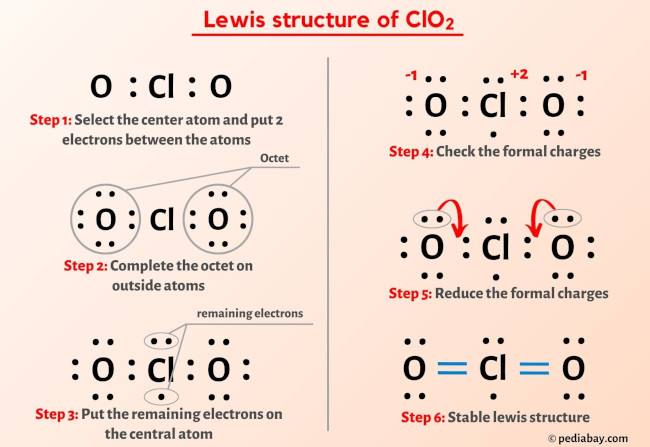So you have seen the above image by now, right?

Let me explain the above image in short

ClO2 lewis structure has a Chlorine atom (Cl) at the center which is surrounded by two Oxygen atoms (O). There are 2 double bonds between the Chlorine atom (Cl) and each Oxygen atom (O). There are 2 lone pairs on both the Oxygen atoms (O) and 1 lone pair & 1 unpaired electron on the Chlorine atom (Cl).

If you haven’t understood anything from the above image of ClO2 lewis structure, then just stick with me and you will get the detailed step by step explanation on drawing a lewis structure of ClO2.

So let’s move to the steps of drawing the lewis structure of ClO2 molecule.

## Steps of drawing ClO2 lewis structure

### Step 1: Find the total valence electrons in ClO2

In order to find the total valence electrons in ClO2, first of all you should know the valence electrons present in chlorine atom as well as oxygen atom.
(Valence electrons are the electrons that are present in the outermost orbit of any atom.)

Here, I’ll tell you how you can easily find the valence electrons of chlorine as well as oxygen using a periodic table.

Total valence electrons in ClO2 molecule

→ Valence electrons given by chlorine atom:Chlorine is group 17 element on the periodic table.  Hence the valence electrons present in chlorine is 7.You can see the 7 valence electrons present in the chlorine atom as shown in the above image.

→ Valence electrons given by oxygen atom:Oxygen is group 16 element on the periodic table.  Hence the valence electrons present in oxygen is 6.You can see the 6 valence electrons present in the oxygen atom as shown in the above image.

Hence,

Total valence electrons in ClO2 = valence electrons given by 1 chlorine atom + valence electrons given by 2 oxygen atoms = 7 + 6(2) = 19.

### Step 2: Select the central atom

For selecting the center atom, you have to remember that the atom which is less electronegative remains at the center.

Now here the given molecule is ClO2 and it contains chlorine atom (Cl) and oxygen atoms (O).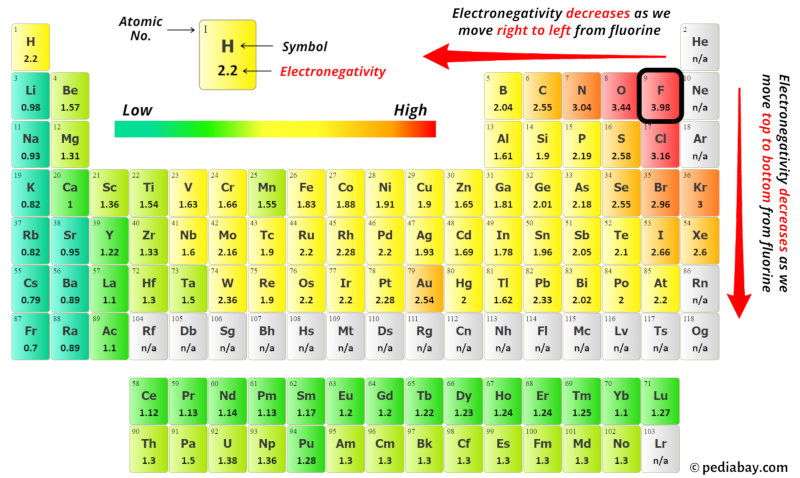You can see the electronegativity values of chlorine atom (Cl) and oxygen atom (O) in the above periodic table.

If we compare the electronegativity values of chlorine (Cl) and oxygen (O) then the chlorine atom is less electronegative.

So here the chlorine atom (Cl) is the center atom and the oxygen atoms (O) are the outside atoms.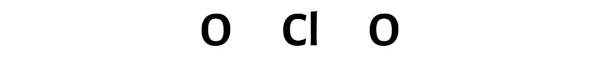Step 3: Connect each atoms by putting an electron pair between them

Now in the ClO2 molecule, you have to put the electron pairs between the chlorine atom (Cl) and oxygen atoms (O).This indicates that the chlorine (Cl) and oxygen (O) are chemically bonded with each other in a ClO2 molecule.

Step 4: Make the outer atoms stable. Place the remaining valence electrons pair on the central atom.

Now in this step, you have to check the stability of the outer atoms.

Here in the sketch of ClO2 molecule, you can see that the outer atoms are oxygen atoms.

These outer oxygen atoms are forming an octet and hence they are stable.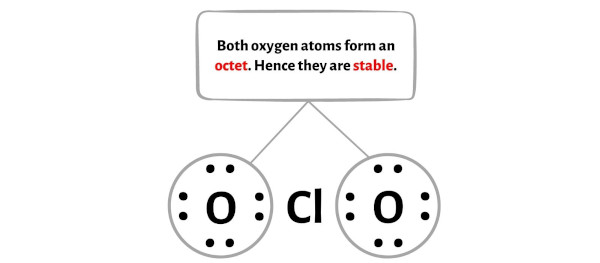Also, in step 1 we have calculated the total number of valence electrons present in the ClO2 molecule.

The ClO2 molecule has a total 19 valence electrons and out of these, only 16 valence electrons are used in the above sketch.

So the number of electrons which are left = 19 – 16 = 3.

You have to put these 3 electrons (i.e 1 electron pair and 1 unpaired electron) on the central chlorine atom in the above sketch of ClO2 molecule.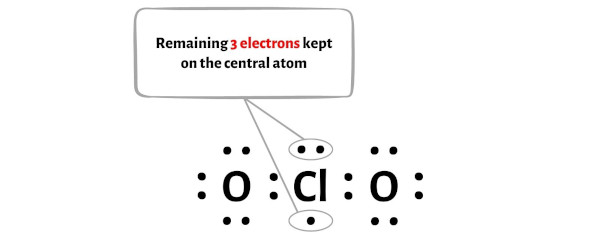Now let’s proceed to the next step.

### Step 5: Check the stability of lewis structure

Now you have come to the final step in which you have to check the stability of lewis structure of ClO2.

The stability of lewis structure can be checked by using a concept of formal charge.

In short, now you have to find the formal charge on chlorine (Cl) atom as well as oxygen (O) atoms present in the ClO2 molecule.

For calculating the formal charge, you have to use the following formula;

Formal charge = Valence electrons – (Bonding electrons)/2 – Nonbonding electrons

You can see the number of bonding electrons and nonbonding electrons for each atom of ClO2 molecule in the image given below.For Chlorine (Cl) atom:
Valence electron = 7 (because chlorine is in group 17)
Bonding electrons = 4
Nonbonding electrons = 3

For Oxygen (O) atom:
Valence electrons = 6 (because oxygen is in group 16)
Bonding electrons = 2
Nonbonding electrons = 6

 Formal charge = Valence electrons – (Bonding electrons)/2 – Nonbonding electrons Cl = 7 – 4/2 – 3 = +2 O = 6 – 2/2 – 6 = -1

From the above calculations of formal charge, you can see that the chlorine (Cl) atom has +2 charge and both the oxygen (O) atom has -1 charge.Because of this reason, the above obtained lewis structure of ClO2 is not stable.

So we have to minimize these charges by shifting the electron pairs towards the chlorine atom.After shifting the electron pair from oxygen atom to chlorine atom, the lewis structure of ClO2 becomes more stable.In the above lewis dot structure of ClO2, you can also represent each bonding electron pair (:) as a single bond (|). By doing so, you will get the following lewis structure of ClO2.I hope you have completely understood all the above steps.

For more practice and better understanding, you can try other lewis structures listed below.

Try (or at least See) these lewis structures for better understanding:

 BeCl2 Lewis Structure CH3COO- Lewis Structure Acetone (C3H6O) Lewis Structure POCl3 Lewis Structure HNO2 Lewis Structure HCO3- Lewis Structure

Jay is an educator and has helped more than 100,000 students in their studies by providing simple and easy explanations on different science-related topics. He is a founder of Pediabay and is passionate about helping students through his easily digestible explanations.